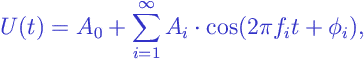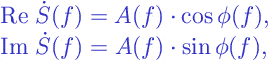# Fourier Spectrum

Fourier Spectrum:

Any continuous functiondefined on a finite intervalof lengthcan be represented as a weighted sum of cosine functions with periods:where

•is the frequency of the i-th Fourier component;
•is the amplitude of the i-th component;
•is the phase of the i-th component.

The functiondescribing the dependence of the amplitudeon the frequencyin the above expression is called the amplitude spectrum of the function.

The functiondescribing the dependence of the phaseon the frequencyis called the phase spectrum of the function.

Thus, the Fourier spectrum of a functionis represented by two functions of the frequency– the amplitude spectrumand the phase spectrum. These two are often combined into a single complex-valued function:where

•is the real part of a complex number;
•is the imaginable part of a complex number;

The concept of the spectrum plays an important role in signal processing , time series analysis , spectral analysis .

Browse Other Glossary Entries

## Test Yourself

Planning on taking an introductory statistics course, but not sure if you need to start at the beginning? Review the course description for each of our introductory statistics courses and estimate which best matches your level, then take the self test for that course. If you get all or almost all the questions correct, move on and take the next test.

### Data Analytics

Considering becoming adata scientist, customer analyst or our data science certificate program?

Analytics Quiz

Statistics Quiz

### Statistics

Looking at statistics for graduate programs or to enhance your foundational knowledge?

Statistics 1 Quiz

Regression Quiz

Regression Quiz

### Biostatistics

Entering the biostatistics field? Test your skill here.

Biostatistics Quiz

Statistics 2 Quiz

### Stay Informed

Our Blog

Read up on our latest blogs

Certificates

Courses

Find the right course for you